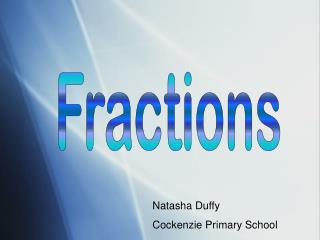DownloadDownload PresentationFractions

# Fractions

Télécharger la présentation## Fractions

- - - - - - - - - - - - - - - - - - - - - - - - - - - E N D - - - - - - - - - - - - - - - - - - - - - - - - - - -
##### Presentation Transcript

1. Fractions Natasha Duffy Cockenzie Primary School

2. FRACTIONS Learning Outcome I can… * explain what a fraction is. * explain what the denominator of a fraction is and find the denominator of a fraction. * explain what the numerator of a fraction is and find the numerator of a fraction. * write a fraction.

3. WHAT IS A FRACTION? • When an object is divided into a number of equal parts then each part is called a fraction

4. 2 numerator denominator 3

5. 1 2 Denominator The denominator says how many equal parts in the whole object 2 equal parts

6. 1 How many equal parts are there in this shape? What is the denominator of this fraction?

7. 1 1 1 1 Find the denominators of these fractions

8. 1 6 1 3 1 4 1 10 Draw what you think these fractions look like.

9. 1 2 So, the number on the bottom of a fraction tells us… ? (Find a partner and discuss what you think the answer is)

10. 1 2 numerator The numerator says how many parts in the fraction 1 part is coloured in

11. 4 How many equal parts are coloured in in this shape? What is the numerator of this fraction?

12. 4 3 8 8 Find the numerator for these fractions

13. 2 6 1 3 3 4 9 10 Draw what you think these fractions look like.

14. 1 2 So, the number on the top of a fraction tells us… ? (Find a partner and discuss what you think the answer is)

15. 2 The numerator tells us… The denominator tells us… 3

16. Find the fraction of these shapes